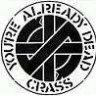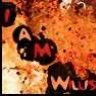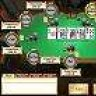# Calculating simple pot odds#### TheNoob

##### Visionary
(I copied this from my post in the ring game hand analysis. If it belongs anywhere, I guess it's here. Can you help me to understand calculating simple pot odds?).

I found this somewhere in the interweb to explain pot odds:

The pot is \$30, and the cost of the call is \$10. The pot odds in this situation are 30:10, or 3:1 when reduced. To get the percentage, we add 3 plus 1 get a sum of 4. Now we divide 1 by 4, giving us 0.25, or 25 percent.

So with my example it would be:

The pot is \$1.95, and the cost of the call is \$1.45. The pot odds in this situation are 1.95:1.45, or 1.35:1 when reduced. To get the percentage, we add 1.35 plus 1 get a sum of 2.35. Now we divide 1 by 2.35, giving us 0.42, or 42 percent.

Where the heck is my error here?

Part of my problem here if I can't do simple math and understand simple pot odds.#### rindhoops

##### Guest
I'm as thick as 2 very short planks when it comes to math so I use
Holdem Indicator, it gives instant pot odds and lots of other info.

see my in-depth review of it (somewhere on this site)#### TheNoob

##### Visionary
I'm as thick as 2 very short planks when it comes to math so I use
Holdem Indicator, it gives instant pot odds and lots of other info.

see my in-depth review of it (somewhere on this site)

<LI class=main>Pot Odds: Pot odds is simply a ratio of the amount of money in the pot compared with how much money it takes to call. The higher the ratio, the better your pot odds are. If there are \$12 in the pot and it costs \$4 to call then you are getting 3:1 odds ( winnings : "cost to stay in"). This can be translated to a percentage, representing the size of your contribution in the new pot. It will be 25% in the above example.
This the same as the first example in my post.

Why the heck doesn't my example work then?:

The pot is \$1.95, and the cost of the call is \$1.45. The pot odds in this situation are 1.95:1.45, or 1.35:1 when reduced. To get the percentage, we add 1.35 plus 1 get a sum of 2.35. Now we divide 1 by 2.35, giving us 0.42, or 42 percent.

Why I'm confused is because the \$1.95 pot and the \$1.45 to call has already been expressed as (1.95+1.45)/1.45 = 2.3:1 = 30%#### c9h13no3

##### Is drawing with AK
Its just in how you're calculating things.

There *isn't* just \$1.95 in the pot in the thread you posted. There is 1.95+1.45 or \$3.40.

For another example, if there is 10\$ in the pot, and someone bets \$5, then if you are next to act, the pot is \$15 and it costs \$5 for you to call.

So simply, to get the odds expressed as a ratio, you divide the two.

15/5 = 3, or 3:1.#### TheNoob

##### Visionary
Its just in how you're calculating things.

There *isn't* just \$1.95 in the pot in the thread you posted. There is 1.95+1.45 or \$3.40.

For another example, if there is 10\$ in the pot, and someone bets \$5, then if you are next to act, the pot is \$15 and it costs \$5 for you to call.

So simply, to get the odds expressed as a ratio, you divide the two.

15/5 = 3, or 3:1.

Ok, that's where I got it wrong.

With the way it's presented above, it would be:

The pot is \$3.40, and the cost of the call is \$1.45. The pot odds in this situation are 3.40:1.45, or 2.35:1 when reduced. To get the percentage, we add 2.35 plus 1 get a sum of 3.35. Now we divide 1 by 3.35, giving us 0.298, or 30 percent.

I'm better now .....

Thanks again c9.#### I Am Willis

##### Guest
BODMAS my sir BODMAS#### RickH2005

##### Legend
Pot Odds?

I just keep it real simple!If there is ALOT of money in the pot and to call is a little money, I call!Poker Odds - Pot & Implied Odds - Odds Calculator